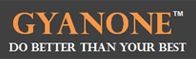# If a and b are both positive integers (Number Properties)

If a and b are both positive integers, is b^(a+1) - b(a^b) odd?

(1) a + (a + 4) + (a - 8) + (a + 6) + (a - 10) is odd
(2) b^3 + 3b^2 + 5b + 7 is odd

Explanation:

The trick to solving this question is to remember that for positive integers:

(a) Even^(positive integer) = even
(b) Odd^(positive integer) = odd
(c) even x even = even x odd = even
(d) even – even = even
(e) odd – odd = even
(f) even – odd = odd

Using statement (1):

5a-8 is odd
=> a is odd

Now evaluating b^(a+1) - ba^b, we get b^even -b*odd
If b is even, the expression is even^even - even = even
If b is odd, the expression is odd^even - odd = even
Therefore in either case, we can say that the expression is not odd. Sufficient.

Using statement (2):

b^3 + 3b^2 + 5b + 7 is odd
=> b^3 + 3b^2 + 5b is even
=> b is even (because if b is odd, the expression would be odd + odd + odd = odd)

Now evaluating b^(a+1) - b(a^b)
we get even^(a+1) - even*a^odd
= even - even*a^odd
= even
Therefore statement (2) is sufficient.GyanOne is an educational services firm focused on premium GMAT coaching, application advisory (with a focus on ISB applications), and interview preparation for B-school admissions. GyanOne operates in the New Delhi region of India and has top global B-school alumni with a minimum score of 770 on the GMAT, as instructors. All GyanOne counsellors are professionally trained top B-school alumni with an experience of 100+ MBA applications behind them.1. Complete GMAT RC Questions in less than 1 minute and 50 seconds
3. Take Notes Effectively
4. Collect and Interpret Facts
5. Speed up Summary Creation
6. Remember Information
7. Question the Author

12. Learn to Answer GMAT organization of passage Question
13. Learn to identify the style/tone or attitude of the author

Mastering GMAT Critical ReasoningAfter you read F1GMAT’s Mastering GMAT Critical Reasoning Guide, you will learn:

How to overcome flawed thinking in GMAT Critical Reasoning?

How to spot Inconsistencies in Arguments

How to eliminate out of scope answer choices using Necessary and Sufficient Conditions

How to Paraphrase GMAT Critical Reasoning Question

How to Answer Assumption Question Type

How to Answer Conclusion Question Type

How to Answer Inference Question Type

How to Answer Strengthen Question Type

How to Answer Weaken Question Type

How to Answer bold-faced and Summary Question Types

How to Answer Parallel Reasoning Questions

How to Answer the Fill in the Blanks Question

Get F1GMAT's Newsletters (Best in the Industry)

• Ranking Analysis
• Post-MBA Salary Trends
• Post-MBA Job Function & Industry Analysis
• Post-MBA City Review
• MBA Application Essay Tips
• School Specific Essay Tips
• GMAT Preparation Tips